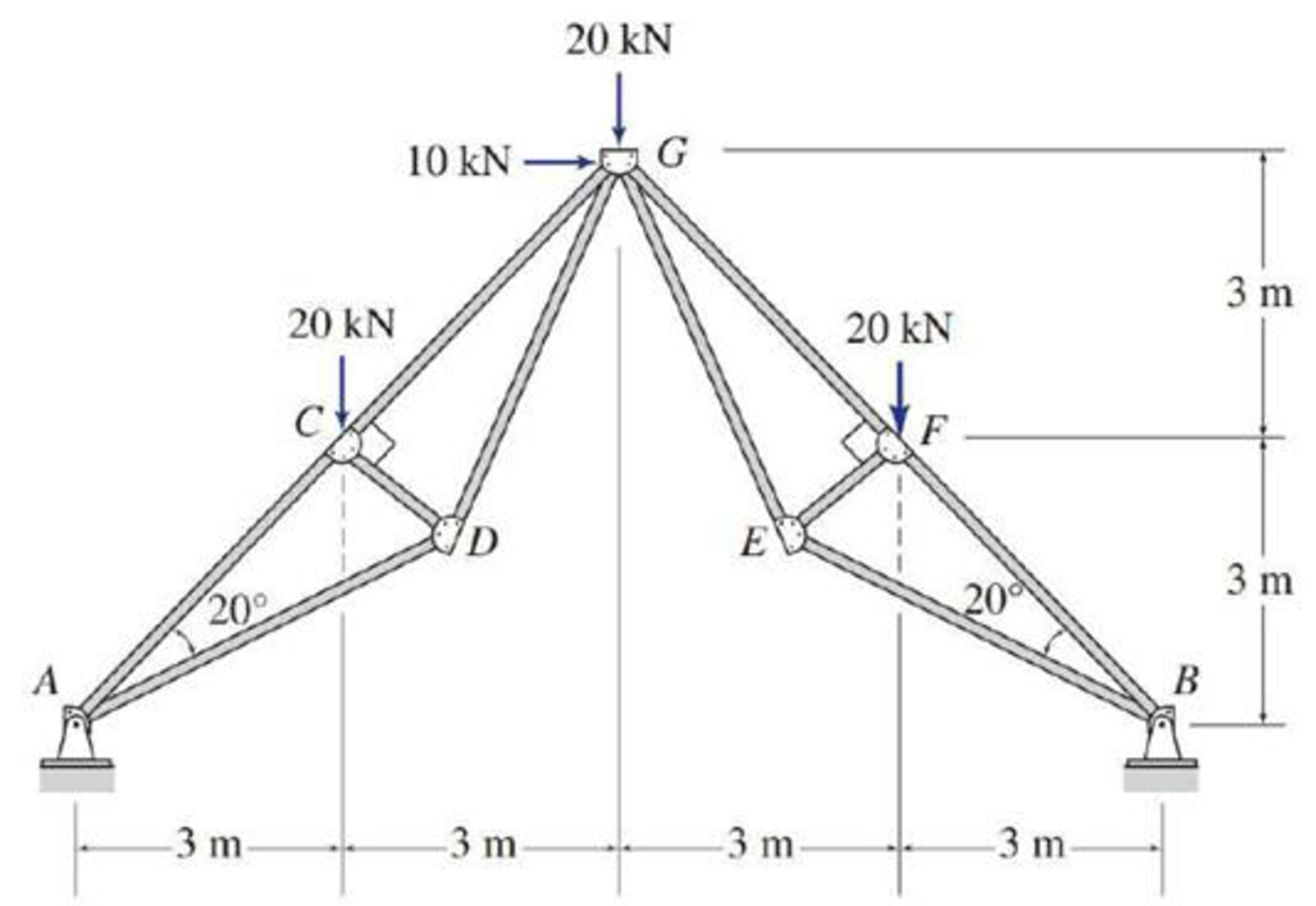# 4.6 through 4.28 Determine the force in each member of the truss shown by the mehod of joints. FIG. P4.27

#### Solutions

Chapter
Section
Chapter 4, Problem 27P
Textbook Problem
98 views

## 4.6 through 4.28 Determine the force in each member of the truss shown by the mehod of joints.FIG. P4.27

To determine

Find the forces in the members of the truss by the method of joints.

### Explanation of Solution

Given information:

Apply the sign conventions for calculating reactions, forces and moments using the three equations of equilibrium as shown below.

• For summation of forces along x-direction is equal to zero (Fx=0), consider the forces acting towards right side as positive (+) and the forces acting towards left side as negative ().
• For summation of forces along y-direction is equal to zero (Fy=0), consider the upward force as positive (+) and the downward force as negative ().
• For summation of moment about a point is equal to zero (Matapoint=0), consider the clockwise moment as negative and the counter clockwise moment as positive.

Method of joints:

The negative value of force in any member indicates compression (C) and the positive value of force in any member indicates Tension (T).

Calculation:

Consider the forces in the member AC, CG, BF, FG, AD, DG, BE, EG, CD, FE are FAC,FCG,FBF,FFG,FAD,FDG,FBE,FEG,FCD,FFE.

Show the free body diagram of the truss as shown in Figure 1.

Refer Figure 1.

Consider the horizontal and vertical reactions at A are Ax and Ay.

Consider the horizontal and vertical reactions at B are Bx and By.

Take the sum of the forces in the vertical direction as zero. Then,

Fy=0Ay+By=20+20+20Ay+Dy=60        (1)

Take the sum of the moments at A is zero.

MA=0(20×3)(10×6)(20×6)(20×9)+By×12=06060120180+By×12=0By×12=420By=35kN

Substitute 35kN for By in Equation (1).

Ay+35=60Ay=25kN

Take the sum of the forces in the horizontal direction as zero.

Fx=0Ax+10Bx=0        (2)

Consider the portion of the truss to the left of the section passing through G.

Take sum of the moments about G is zero.

MG=0Ay×6+Ax×6+20×3=025×6+Ax×6+20×3=0Ax=906Ax=15kN

Substitute 15kN for Ax in Equation (1).

15+10Bx=10Bx=25kN

Calculate the value of the angle θ as follows:

tanθ=33θ=tan1(33)θ=45°

Show the joint A as shown in Figure 2.

Refer Figure 2.

Find the forces in the members AC and AD.

For Equilibrium of forces,

Solve Equation (3) and (4).

Show the joint C as shown in Figure 3.

Refer Figure 3.

Find the forces in the members CD and CG.

For Equilibrium of forces,

Fx=0FCA+FCG20cos45°=0(47.71)+FCG20cos45°=0(47

### Still sussing out bartleby?

Check out a sample textbook solution.

See a sample solution

#### The Solution to Your Study Problems

Bartleby provides explanations to thousands of textbook problems written by our experts, many with advanced degrees!

Get Started

Find more solutions based on key concepts
Compute the nominal flexural strength of the composite beam in Problem 9.1-2. Use Fy=50 ksi.

Steel Design (Activate Learning with these NEW titles from Engineering!)

A jet plane taking off creates a noise with a magnitude of approximately 125 dB. What is the magnitude of the p...

Engineering Fundamentals: An Introduction to Engineering (MindTap Course List)

Upright drill presses are available in _______ and __________ models.

Precision Machining Technology (MindTap Course List)

What is strategy?

Management Of Information Security

What are Pipkins three categories of incident indicators?

Principles of Information Security (MindTap Course List)

What were ARPAs original goals? (56)

Enhanced Discovering Computers 2017 (Shelly Cashman Series) (MindTap Course List)

What emergency steps should be taken to treat burns?

Welding: Principles and Applications (MindTap Course List)

If your motherboard supports ECC DDR3 memory, can you substitute non-ECC DDR3 memory?

A+ Guide to Hardware (Standalone Book) (MindTap Course List)